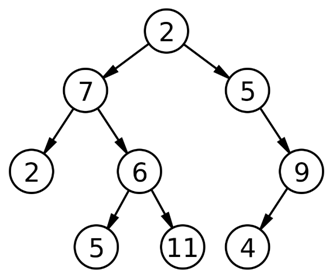# Leftmost and Rightmost Nodes of Binary Tree

In this article, we are going to see how to print only the leftmost and rightmost nodes? This problem has been featured in Amazon.
Submitted by Radib Kar, on February 22, 2019

Problem statement:

Given a Binary Tree, Print the corner nodes at each level. The node at the leftmost and the node at the rightmost.

Example:For this tree output will be: 2 7 5 2 9 5 4

Solution:

Of course the solution includes level order traversal.

Algorithm:

FUNCTION printCorner (root): prints corner nodes (leftmost, rightmost) of the tree

Prerequisite: Queue q, input binary tree root

```FUNCTION printCorner(Node *root)
1.  Declare a queue to store pointer to tree nodesq;
2.  Declare store=0 which will print the rightmost node;
3.  Declare temp to store DeQueued element from queue ;
4.  Declare count=0 which will help to print the leftmost node;
5.  Print the root->data first
6.  IF (root->left)
EnQueue (q,root->left);
IF (root->right)
EnQueue (q,root->right);
EnQueue (q, NULL); //to indicate end of first level (root only)
7.  While(q is not empty){
temp=DeQueue(q);
Increment count;
//temp is not NULL && temp is pointer to the first node (leftmost)
IF(temp && count==1)
Print temp->data;
ELSE IF(temp) //for other nodes rather than the leftmost one
store=temp->data; //update store each time with latest node value
END IF-ELSE
IF (temp==NULL) //end of previous level
IF (q is not empty)
EnQueue(q,NULL);
END IF
count=0; //re-define countas 0 for next level
Print store; //store consist of rightmost of last level node value
ELSE
IF(temp->left is not NULL)
EnQueue(q, temp->left);
IF(temp->right is not NULL)
EnQueue(q, temp->right);
END IF-ELSE
END WHILE
END FUNCTION
```

Example with explanation:N.B: The nodes are represented by their respective values.

For the above example:

Nodes are represented with their respective values for better understanding.

```In the main we call printCorner (2)
----------------------------------------------------

printCorner (2)
store=0
count=0
Print root//2
root->left is not NULL
q.push(root->left)//7
root->right is not NULL
q.push(root->right)//5
q.push(NULL)
----------------------------------------------------

1st iteration:
q is not empty
temp= q.front()=7
q.pop()
Queue status:
5 NULL
Count=1
Temp not NULL and count is 1
Print 7
7->left not NULL
q.push (7->left) //2
7->right not NULL
q.push(7->right) //6
Queue status:
5 NULL 2 6
----------------------------------------------------

2nd iteration:
q is not empty
temp= q.front()=5
q.pop()
count=2; //incremented
Queue status:
2 6
temp not NULL and count is not 1
Update store with temp->data// store=5
5->leftNULL
5->right not NULL
q.push(5->right) //9
Queue status:
NULL 2 6 9
----------------------------------------------------

3rd iteration:
q is not empty
temp= q.front()=NULL
q.pop()
count=3 //incremented
Queue status:
2 6 9
Temp is NULL
Print store // 5
Count re-initialized to 0
q is not empty
q.push(NULL)
Queue status:
2 6 9 NULL
----------------------------------------------------

4th iteration:
q is not empty
temp= q.front()=2
q.pop()
count=1; //incremented
temp is not NULL && count is 1
Print temp//2
Queue status:
6 9 NULL
2->left NULL
2->right NULL

Queue status:
6 9 NULL
----------------------------------------------------

5th iteration:
q is not empty
temp= q.front()=6
q.pop()
count=2; //incremented
temp is not NULL , but count not 1
store=6
Queue status:
9 NULL
6->left  not NULL
q.push(6->left) //5
6->right not NULL
q.push(6->left) //11
Queue status:
9 NULL 5 11
----------------------------------------------------

6th iteration:
q is not empty
temp= q.front()=9
q.pop()
count=3; //incremented
temp is not NULL , but count not 1
store=9
Queue status:
NULL 5 11
9->left  not NULL
q.push(9->left) //4
9->right NULL
Queue status:
NULL 5 11 4
----------------------------------------------------

7th iteration:
q is not empty
temp= q.front()=NULL
q.pop()
Queue status:
5 11 4
Count=4//incremented
Temp is NULL
Print store // 9
Count re-initialized to 0
q is not empty
q.push(NULL)
Queue status:
5 11 4 NULL
----------------------------------------------------

8th iteration:
q is not empty
temp= q.front()=5
q.pop()
Queue status:
11 4 NULL
Count=1; //incemented
Temp is not NULL and count is 1
Print temp->data //5
5->left   NULL
5->right NULL

Queue status:
11 4 NULL
----------------------------------------------------

9th iteration:
q is not empty
temp= q.front()=11
q.pop()
Queue status:
4 NULL
Count=2; //incemented
temp is not NULL and count is not 1
store= temp->data //11
11->left   NULL
11->right NULL

Queue status:
4 NULL
----------------------------------------------------

10th iteration:
q is not empty
temp= q.front()=4
q.pop()
Queue status:
NULL
Count=4; //incemented
temp is not NULL and count is not 1
store= temp->data //4
4->left   NULL
4->right NULL
----------------------------------------------------

11th iteration:
q is not empty
temp= q.front()=NULL
q.pop()
Queue status:
Empty
Count=5//incremented
Temp is NULL
Print store // 5
Count re-initialized to 0
qis empty
Queue status:
Empty
----------------------------------------------------
Iteration ends

Queue status:
Empty
Output:
2 7 5 2 9 5 4
```

C++ implementation:

```#include <bits/stdc++.h>
using namespace std;

// tree node is defined
class Node{
public:
int data;
Node *left;
Node *right;
};

void printCorner(Node *root){
queue<Node*> q;
int store=0;
Node* temp;
int count=0;

cout<<root->data<<"\n";
if(root->left)
q.push(root->left);
if(root->right)
q.push(root->right);
q.push(NULL);
while(!q.empty()){
temp=q.front();
q.pop();
count++;
if(temp && count==1)
cout<<temp->data<<" ";
else if(temp)
store=temp->data;
if(temp==NULL){
count=0;
cout<<store<<"\n";
if(!q.empty()){
q.push(NULL);
}
}
else{
if(temp->left)
q.push(temp->left);
if(temp->right)
q.push(temp->right);
}
}
}

// creating new node
Node* newnode(int data)
{
Node* node = (Node*)malloc(sizeof(Node));
node->data = data;
node->left = NULL;
node->right = NULL;
return(node);
}

int main() {
//**same tree is builted as shown in example**
cout<<"same tree is builted as shown in example\n";
Node *root=newnode(2);

root->left= newnode(7);
root->right= newnode(5);
root->right->right=newnode(9);
root->right->right->left=newnode(4);
root->left->left=newnode(2);
root->left->right=newnode(6);
root->left->right->left=newnode(5);
root->left->right->right=newnode(11);

cout<<"Printing the right most & left most nodes of binary tree...:"<<endl;
printCorner(root);

return 0;
}
```

Output

```same tree is builted as shown in example
Printing the right most & left most nodes of binary tree...:
2
7 5
2 9
5 4
```